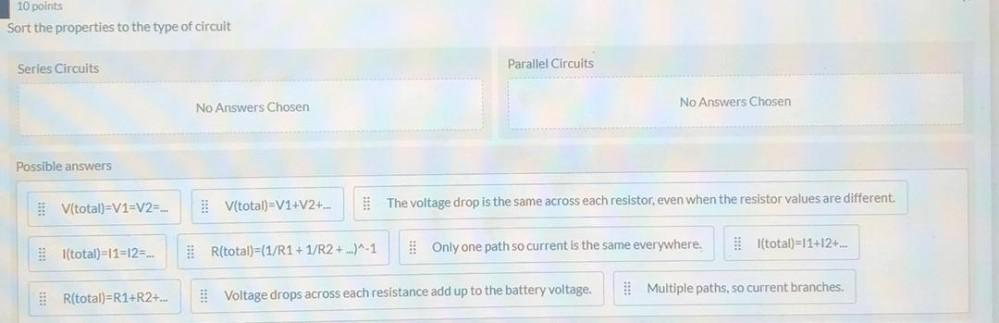Question:

# 10 points Sort the properties to the type of circuit Series Circuits Parallel Circuits No Answers Chosen No Answers Chosen Possi10 points Sort the properties to the type of circuit Series Circuits Parallel Circuits No Answers Chosen No Answers Chosen Possible answers V(total)=V1=V2=... V(total)=V1+V2+... The voltage drop is the same across each resistor, even when the resistor values are different. I(total)=11=12=... l(total)=11+12+... R(total)=(1/R1+1/R2 + ...)^-1 Only one path so current is the same everywhere. R(total)=R1+R2+... Voltage drops across each resistance add up to the battery voltage. Multiple paths, so current branches.